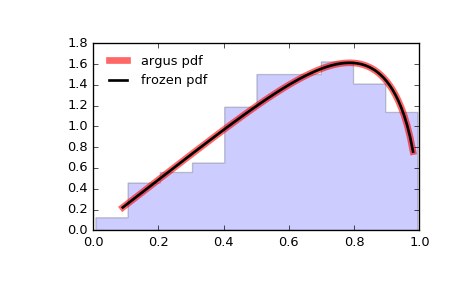# scipy.stats.argus¶

scipy.stats.argus = <scipy.stats._continuous_distns.argus_gen object>[source]

Argus distribution

As an instance of the rv_continuous class, argus object inherits from it a collection of generic methods (see below for the full list), and completes them with details specific for this particular distribution.

Notes

The probability density function for argus is:

argus.pdf(x, chi) = chi**3 / (sqrt(2*pi) * Psi(chi)) * x * sqrt(1-x**2) * exp(- 0.5 * chi**2 * (1 - x**2))

where:
Psi(chi) = Phi(chi) - chi * phi(chi) - 1/2
with Phi and phi being the CDF and PDF of a standard normal distribution, respectively.


argus takes chi as shape a parameter.

References

 [R555] “ARGUS distribution”, https://en.wikipedia.org/wiki/ARGUS_distribution

The probability density above is defined in the “standardized” form. To shift and/or scale the distribution use the loc and scale parameters. Specifically, argus.pdf(x, chi, loc, scale) is identically equivalent to argus.pdf(y, chi) / scale with y = (x - loc) / scale.

New in version 0.19.0.

Examples

>>> from scipy.stats import argus
>>> import matplotlib.pyplot as plt
>>> fig, ax = plt.subplots(1, 1)


Calculate a few first moments:

>>> chi = 1
>>> mean, var, skew, kurt = argus.stats(chi, moments='mvsk')


Display the probability density function (pdf):

>>> x = np.linspace(argus.ppf(0.01, chi),
...                 argus.ppf(0.99, chi), 100)
>>> ax.plot(x, argus.pdf(x, chi),
...        'r-', lw=5, alpha=0.6, label='argus pdf')


Alternatively, the distribution object can be called (as a function) to fix the shape, location and scale parameters. This returns a “frozen” RV object holding the given parameters fixed.

Freeze the distribution and display the frozen pdf:

>>> rv = argus(chi)
>>> ax.plot(x, rv.pdf(x), 'k-', lw=2, label='frozen pdf')


Check accuracy of cdf and ppf:

>>> vals = argus.ppf([0.001, 0.5, 0.999], chi)
>>> np.allclose([0.001, 0.5, 0.999], argus.cdf(vals, chi))
True


Generate random numbers:

>>> r = argus.rvs(chi, size=1000)


And compare the histogram:

>>> ax.hist(r, normed=True, histtype='stepfilled', alpha=0.2)
>>> ax.legend(loc='best', frameon=False)
>>> plt.show()Methods

 rvs(chi, loc=0, scale=1, size=1, random_state=None) Random variates. pdf(x, chi, loc=0, scale=1) Probability density function. logpdf(x, chi, loc=0, scale=1) Log of the probability density function. cdf(x, chi, loc=0, scale=1) Cumulative distribution function. logcdf(x, chi, loc=0, scale=1) Log of the cumulative distribution function. sf(x, chi, loc=0, scale=1) Survival function (also defined as 1 - cdf, but sf is sometimes more accurate). logsf(x, chi, loc=0, scale=1) Log of the survival function. ppf(q, chi, loc=0, scale=1) Percent point function (inverse of cdf — percentiles). isf(q, chi, loc=0, scale=1) Inverse survival function (inverse of sf). moment(n, chi, loc=0, scale=1) Non-central moment of order n stats(chi, loc=0, scale=1, moments='mv') Mean(‘m’), variance(‘v’), skew(‘s’), and/or kurtosis(‘k’). entropy(chi, loc=0, scale=1) (Differential) entropy of the RV. fit(data, chi, loc=0, scale=1) Parameter estimates for generic data. expect(func, args=(chi,), loc=0, scale=1, lb=None, ub=None, conditional=False, **kwds) Expected value of a function (of one argument) with respect to the distribution. median(chi, loc=0, scale=1) Median of the distribution. mean(chi, loc=0, scale=1) Mean of the distribution. var(chi, loc=0, scale=1) Variance of the distribution. std(chi, loc=0, scale=1) Standard deviation of the distribution. interval(alpha, chi, loc=0, scale=1) Endpoints of the range that contains alpha percent of the distribution

#### Previous topic

scipy.stats.arcsine

scipy.stats.beta